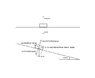# Calculating coefficient of max static friction

arjfsdsr
Hello,

I'm conducting an experiment about how contact force and surface quality affect friction. I know that friction is affected by both, as my data suggests. I measured the static forces of several weights on two different surfaces, and found that indeed the max static force increased as the weights got heavier.

For analysis, I'd like to calculate the coefficients of the max static forces, and I know this is equal to (max static force / normal force), but I'm wondering how to calculate the normal forces of each weight. Is it just equal to the mass of each weight times gravity??

Thanks so much!

jdstokes
Is it just equal to the mass of each weight times gravity??

Yep, consider force balance in the vertical direction.

Archosaur
Is it just equal to the mass of each weight times gravity??
Yes, mass in Kg times gravity (9.81 m/s^2) will give you the force of gravity (in Newtons) that is pulling the object toward the center of the Earth.

If the object is on a level surface, than the force of gravity equal in magnitude to the normal force.

But, if the object is on a slant with an angle "theta" from horizontal, the normal force is the gravitational force times the cosine of "theta". The normal force in this case will be less than it would be on a level surface.

#### Attachments

•normal.jpg
9.3 KB · Views: 427